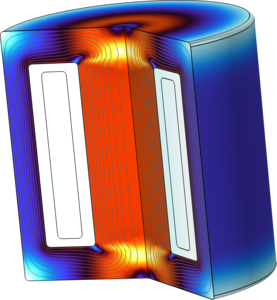# Application Gallery

## Small-Signal Analysis of an Inductor

Application ID: 2128

If an inductor's magnetic material is nonlinear, then the inductance depends on the current passing through it.

This model consists of an inductor with a nonlinear magnetic core, where the small-signal inductance is simulated as a function of current. The model also investigates how the small-signal inductance depends on the DC current.This model example illustrates applications of this type that would nominally be built using the following products: